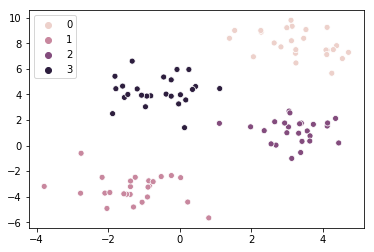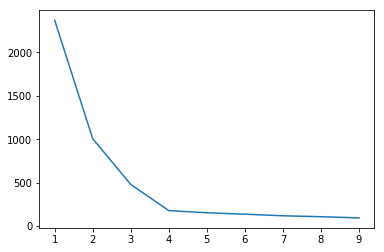In :
#Import sklearn and the makeblobs module for generating dataset
import sklearn
from sklearn.datasets import make_blobs
import pandas as pd
import seaborn as sns

#n_samples = datapoints
#n_features = how many x's
#centers = how many clusters
x,y = make_blobs(n_samples = 100, n_features = 2, centers=4)

#show y values (the generated group)
y

Out:
array([0, 0, 2, 3, 3, 3, 2, 2, 2, 0, 1, 1, 2, 1, 2, 3, 3, 2, 2, 3, 0, 0,
3, 1, 1, 2, 3, 3, 3, 0, 3, 0, 2, 1, 2, 2, 0, 0, 1, 0, 1, 2, 3, 0,
0, 3, 1, 2, 1, 3, 0, 2, 1, 3, 2, 0, 0, 3, 2, 0, 0, 3, 1, 3, 2, 1,
2, 2, 0, 1, 3, 1, 3, 2, 0, 3, 2, 1, 2, 0, 0, 0, 0, 1, 2, 1, 1, 1,
0, 2, 3, 1, 3, 1, 1, 3, 1, 3, 1, 0])

# Plot the data we just generated on a chart, to see what it looks like¶

In :
#x = x, all rows in column1 (feature 1)
#y = x, all rows in column2 (feature 2)
#hue = colour by y (group)

sns.scatterplot(x=x[:, 0], y=x[:, 1], hue=y)

Out:
<matplotlib.axes._subplots.AxesSubplot at 0x1a1687de80># Import k-means & figure out what k should be equal to¶

In :
#Until now, we have just generated a dataset. We know there are 4 clusters because we generated it.
#We won't always already know the clusters in the data
#Now we need to pass it into kmeans to identify the clusters:
from sklearn.cluster import KMeans

#coordinates of the cluster centers
model.cluster_centers_

#higher inertia means the accuracy - higher = worse. Can be controlled by number of clusters
#inertia = amount of spread
model.inertia_

#let's run the algorithm to work out the right number of clusters
inertia=[]
for k in range (1,10):
model = KMeans(n_clusters=k)
model.fit(x)
inertia.append(model.inertia_)


# Plot the inertia values. Once k = 4, it levels out¶

In :
#if we now print those scores, we see that 10 provides the lowest score
inertia

#we can now plot the amount of inertia by number of clusters. 4 is where it flattens (the elbow) We use this one.
sns.lineplot(range(1,10), inertia)

Out:
<matplotlib.axes._subplots.AxesSubplot at 0x1a16d94c88># Run the model for k=4¶

In :
#number of clusters = hyperparameter
model = KMeans(n_clusters=4)
model.fit(x)

Out:
KMeans(algorithm='auto', copy_x=True, init='k-means++', max_iter=300,
n_clusters=4, n_init=10, n_jobs=1, precompute_distances='auto',
random_state=None, tol=0.0001, verbose=0)

# Create output dataframe which maps x to their assigned cluster¶

In :
#create dataframe of input x1 and x2
df = pd.DataFrame({'x1':x[:,0],'x2':x[:,1]})

#generate column for predicted cluster name
y = model.predict(x)
df['cluster'] = y

#show dataframe
df.head()

Out:
x1 x2 cluster
0 1.396995 8.400158 1
1 3.472704 8.397183 1
2 3.047598 1.458551 2
3 -0.025118 3.261093 3
4 -0.375987 4.027548 3
In [ ]:


In [ ]: